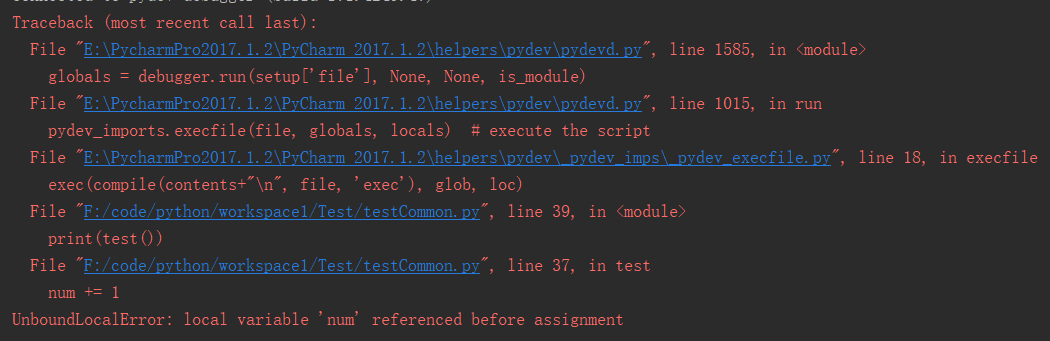# Python UnboundLocalError: local variable ‘num’ referenced before assignment

The source code

``````num = 1

def test():
num += 1
return num

print(test())``````

Error detailsPossible reasons for
Undeclared variables appear in Python, and PY finds the scope of a variable by following a simple rule: If there is an assignment to a variable within a function, the variable is considered local and can be modified normally. However, if the variable is not defined inside the function, and there is no variable scope declaration (to call the external variable), the program cannot find the corresponding variable inside the function, so the error message of undefined variable will appear.
The solution
Declare variables as global variables, use global keywords when calling, and you can access them normally. The correct code is as follows:

``````num = 1

def test():
global num
num = num + 1
return num

print(test())    # output is:2``````